Next: 4.1.2 Modified Two-Level Newton Up: 4.1 The Two-Level Newton Previous: 4.1 The Two-Level Newton

## 4.1.1 Calculation of Conductances

The discretized semiconductor equations are represented by the non-linear equation system f(x) = 0. The solution vector x contains the carrier concentrations, the potential, the contact voltages, and some more quantities depending on the simulation mode. As the contact voltages are the quantities which determine the state of the device, the dependence of the solution vector on the contact voltages is explicitly written in the equation system. For Fig. 4.1 one gets

 f(x(V), V) = 0 (4.3)

By applying Newton's algorithm to (4.3) with V = V0 one gets

 -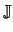kx . u = f(xk, V0)        withkx =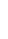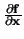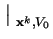(4.4)

The iteration terminates after some convergence criterion is fulfilled. As a next step the contact current I(x, V0) can be calculated. For the calculation of the linearized conductance Geq the chain rule is applied to (4.1) which gives

 Geq =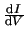=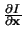.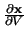+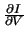(4.5)

where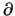I/x andI/V are obtained by symbolic differentiation of the function I(x, V). The quantityx/V can be determined in two different ways. The first approach has been published in . At first, (4.4) is solved until a user-defined convergence criterion is reached. Then a small perturbation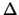V is applied on V0. Using the Taylor expansion of (4.3) with respect to both x and V around the DC solution, f(x0, V0) results in

 f(x0 +x, V0 +V)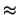f(x0, V0) +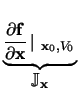.x +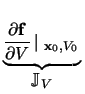.V +...= 0 (4.6)

As f(x0, V0) = 0, the above equation reduces tox .x = -V .V (4.7)

The Jacobian matrixx is the same as for the last iteration of the DC solution and the vectorV .V on the right-hand side normally contains only a few non-zero elements as only the quantities at the boundaries directly depend on V. The solution of the linear system (4.7)x is proportional toV, hencex/V is independent ofV and equal to the limiting case which is the derivative whenV becomes infinitesimally small.=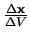= -x-1 .V (4.8)

Another equivalent possibility to calculatex/V is from the total derivative of (4.3) with respect to V which reads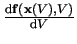=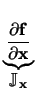.+= 0 (4.9)

and also results in (4.8). To summarize, the equivalent conductance and current are calculated as follows

 Gk + 1eq =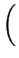-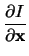.x-1 .V +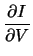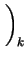(4.10) Ik + 1eq = Ik - Gk + 1eq . Vk (4.11)Next: 4.1.2 Modified Two-Level Newton Up: 4.1 The Two-Level Newton Previous: 4.1 The Two-Level Newton
Tibor Grasser
1999-05-31